1. 矩阵的中心化，归一化，标准化与正规化。
2. 范数，包括向量的范数和矩阵的范数。协方差矩阵。
3. PCA算法与详细证明。
4. 置信区间和置信度

## 矩阵的中心化，归一化，标准化与正规化

### 归一化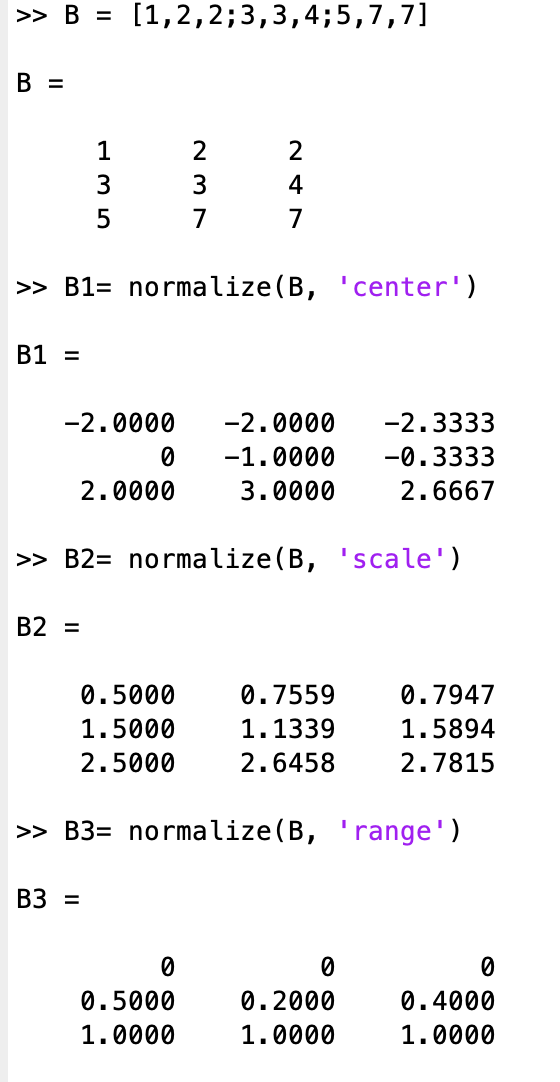### 标准化

$\mu$代表每一列的均值，$\sigma$代表每一列的标准差

### 正规化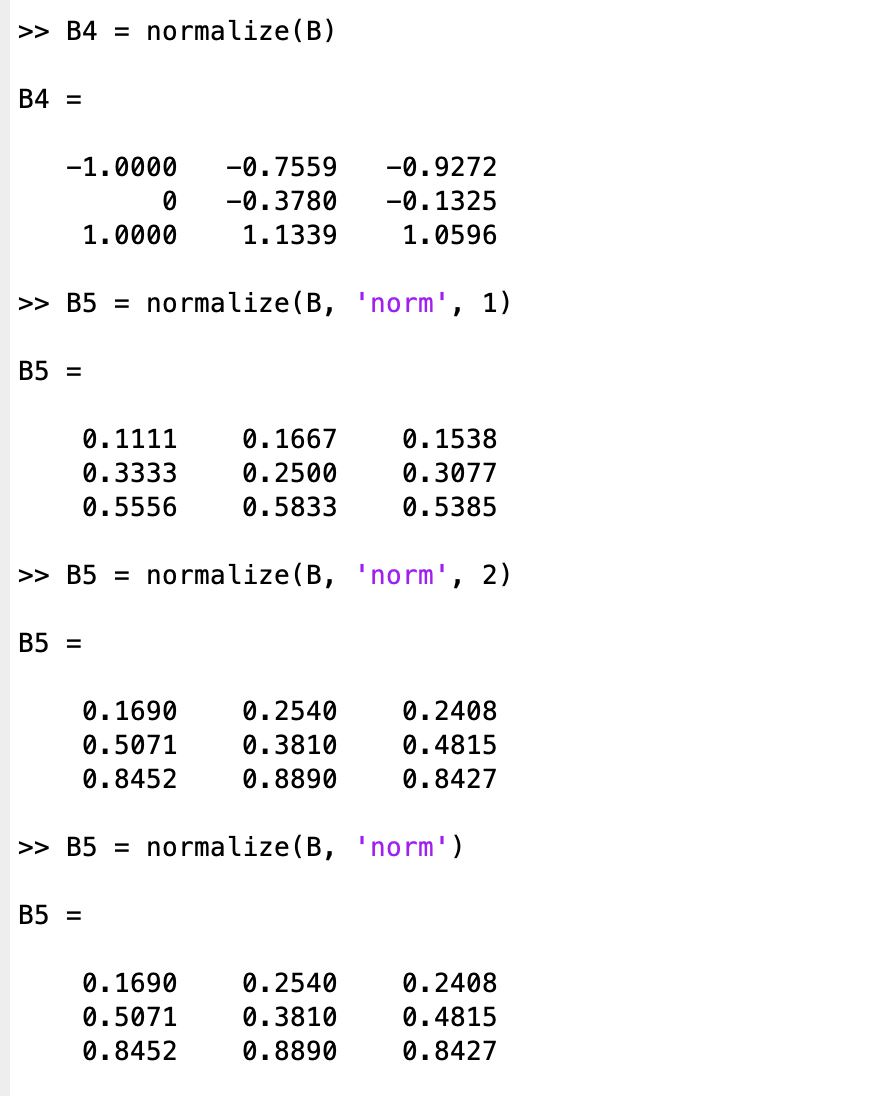## 范数

### 向量的范数

$\infty$范数：$|x|_\infty = \mathop{max}\limits_{i}|x_i|$所有向量元素绝对值中的最大值，matlab调用函数norm(x, inf)
-$\infty$范数：$|x|_{-\infty} = \mathop{min}\limits_{i}|x_i|$所有向量元素绝对值中的最小值，matlab调用函数norm(x, -inf)

p范数：$|x|_p=(\sum\limits_{i=1}^{N}|x_i|^p)^{\frac{1}{p}}$向量元素绝对值的p次方和的1/p次幂，matlab调用函数norm(x, p)

### 矩阵的范数

$\infty$范数：$|A|_{\infty} = \mathop{max}\limits_i\sum\limits_{j=1}^{n}|a_{i,j}|$，行和范数，即所有矩阵行向量绝对值之和的最大值，matlab调用函数norm(A, inf)。
F范数：$|A|_F = \sqrt{\sum\limits_{i=1}^{m}\sum\limits_{j=1}^n|a_{i,j}|^2}$，Frobenius范数，即矩阵元素绝对值的平方和再开平方，matlab调用函数norm(A, ‘fro’)。

## 协方差矩阵

X的每一列减去对应的平均值

X乘以X的转置矩阵，求取协方差矩阵

## PCA降维

### 算法介绍

PCA(Principal Component Analysis)的流程。对于PCA的应用很多，信号数据的去噪处理，图片的压缩等等(把一张长为d宽为n的图片看成是d-dimension的n个数据点组成)。

algorithm PCA (P is a set of n points in d-dimensional space, now we need to convert P into a set P’ of points in a k-dimensional space, $k\leq d$)

algorithm PCA
output: k directional vectors

• shift P such that its geometric mean is at the origin of the data space
• A $\leftarrow$ the co-variance matrix of P
• compute all the d unit eigenvectors
• arrange the eigenvectors in descending order of their eigenvalues
• return the first k eigenvectors $v1,…,vk$

Each point p in P is then converted to a k-dimensional point whose i-th($1\leq i\leq d$)coordinate is $v_i\cdot p$

### 计算样例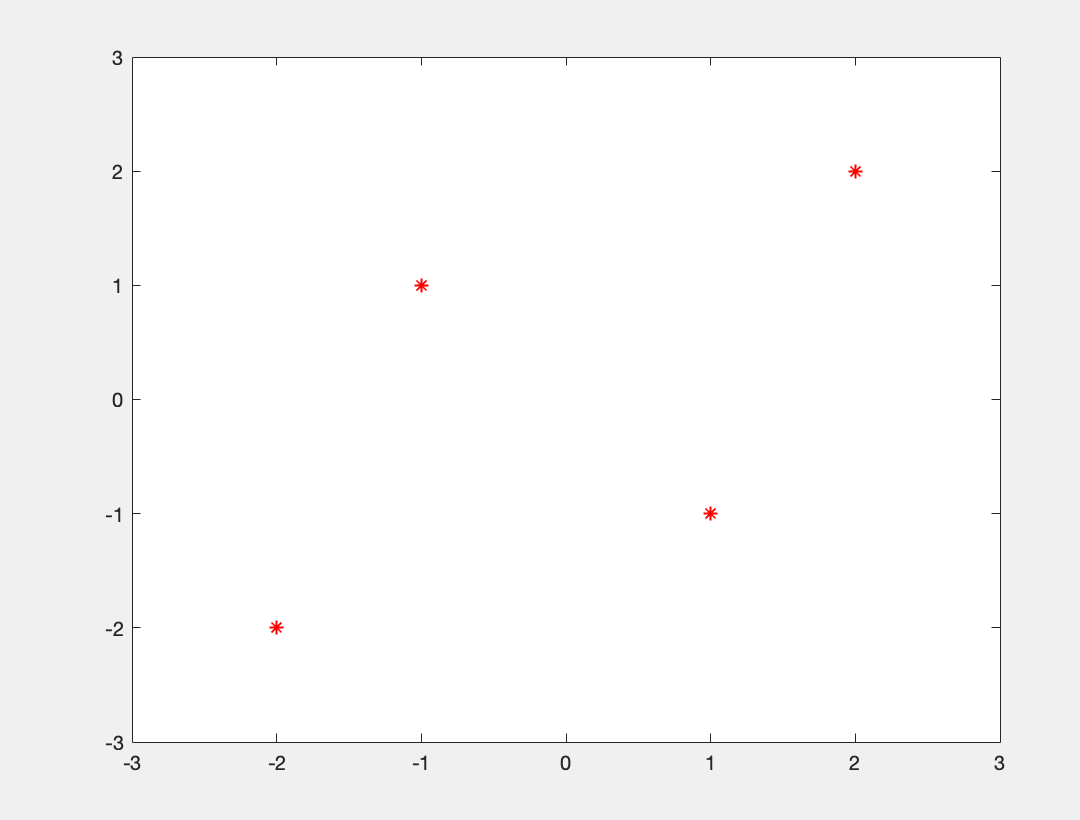$I$是单位矩阵(identity matrix), $I = {\left [ \begin{array}{ccc} 1 & 0 \\ 0 & 1 \end{array} \right ]}$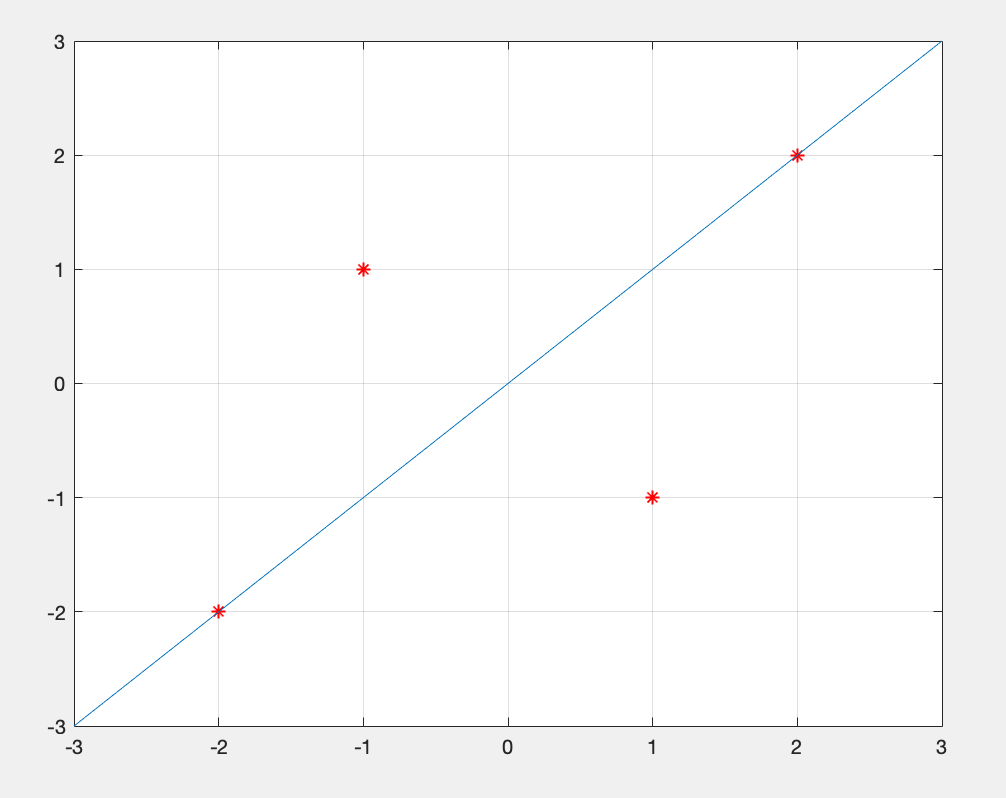### 原理解释

$\overrightarrow{w}$是单位向量，那么我们现在在$\overrightarrow{w^{T}}\overrightarrow{w} = 1$这个constraint下，尽可能提升quality的数值。那么其实上述的问题变成了一个maximize的问题, 利用拉格朗日数乘法构造下面一个式子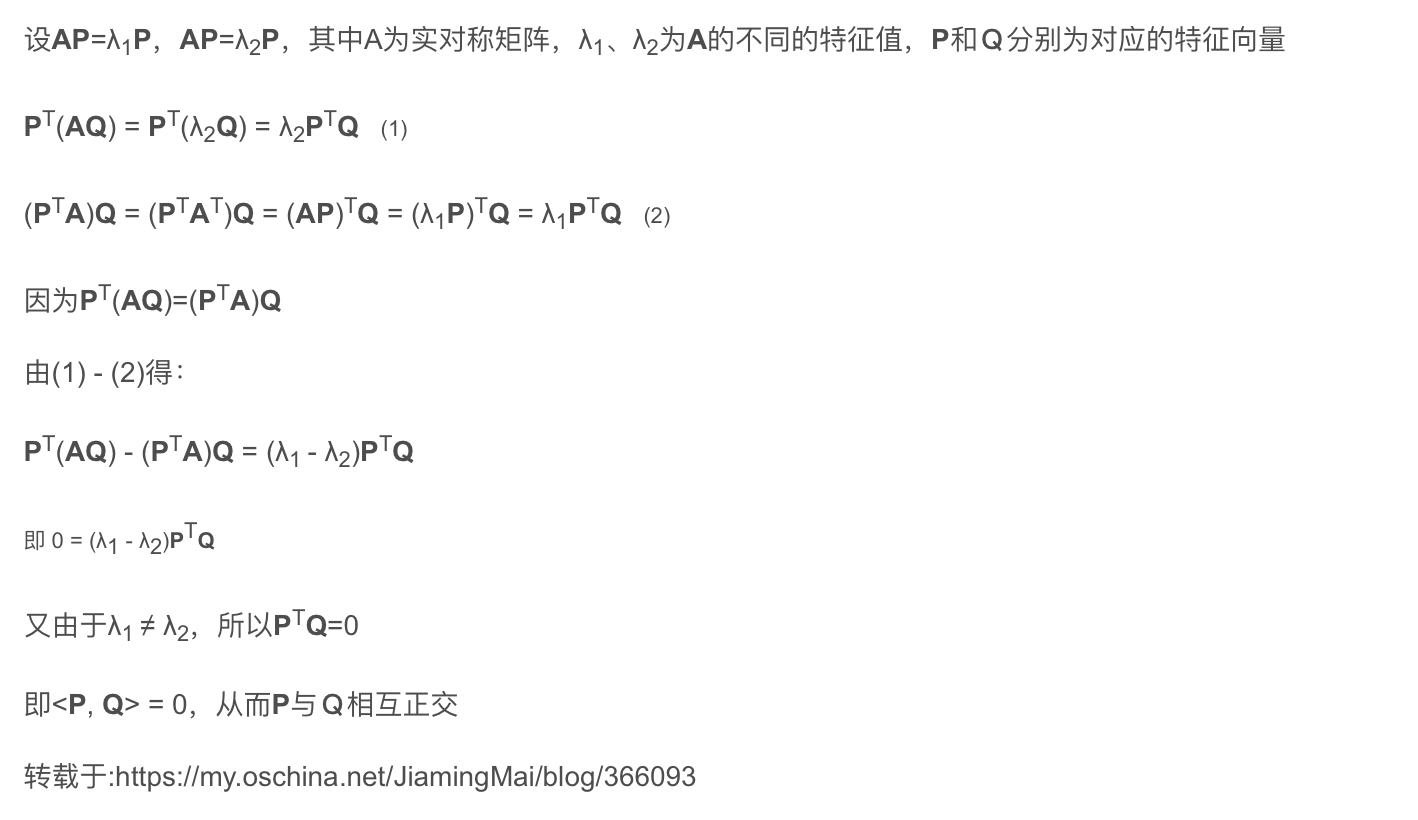### FastMap

Heuristic 1 of FastMap

Assume the vector between the farthest pair of points in P captures a large amount of variance of P.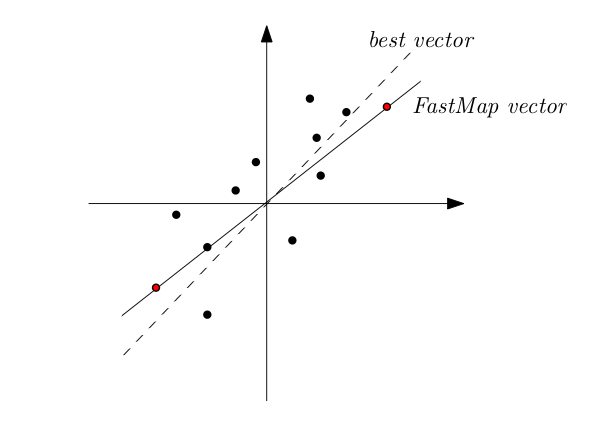Heuristic 2 of FastMap

Use the following algorithm to find the farthest pair of points in P

• $p_1 \leftarrow$ a random point in P
• $p_2 \leftarrow$ farthest point from $p_1$
• $p_1 \leftarrow$ farthest point from $p_2$